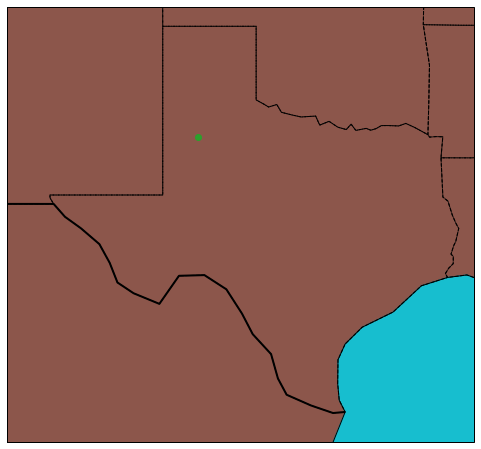# CartoPy## Plotting on a Map with CartoPy

#### Unidata Python Workshop#### Questions¶

1. How do we plot on a map in Python?
2. How do I specify a map projection?
3. How do I tell CartoPy how to reference my data?
4. How do I add map features to a CartoPy plot?

### 1. Basic CartoPy Plotting¶

• High level API for dealing with maps
• CartoPy allows you to plot data on a 2D map.
• Support many different map projections
• Support for shapefiles from the GIS world
In :
# Set things up
%matplotlib inline

# Importing CartoPy
import cartopy.crs as ccrs
import cartopy.feature as cfeature
import matplotlib.pyplot as plt


The simplest plot we can make sets a projection with no parameters. The one below uses the Robinson projection:

In :
# Works with matplotlib's built-in transform support.
fig = plt.figure(figsize=(10, 4))
ax = fig.add_subplot(1, 1, 1, projection=ccrs.Robinson())

# Sets the extent to cover the whole globe
ax.set_global()

ax.stock_img()

Out:
<matplotlib.image.AxesImage at 0x7f3a38dde690>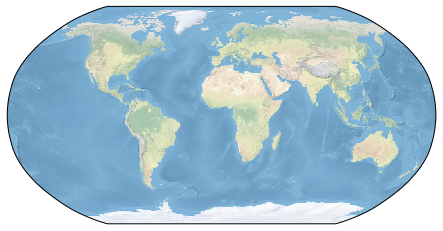We also have fine-tuned control over the globe used in the projection as well as lots of standard parameters, which depend on individual projections:

In :
# Set up a globe with a specific radius
globe = ccrs.Globe(semimajor_axis=6371000.)

# Set up a Lambert Conformal projection
proj = ccrs.LambertConformal(standard_parallels=[25.0], globe=globe)

fig = plt.figure(figsize=(10, 8))
ax = fig.add_subplot(1, 1, 1, projection=proj)

# Sets the extent using a lon/lat box
ax.set_extent([-130, -60, 20, 55])

ax.stock_img()

Out:
<matplotlib.image.AxesImage at 0x7f3a192df550>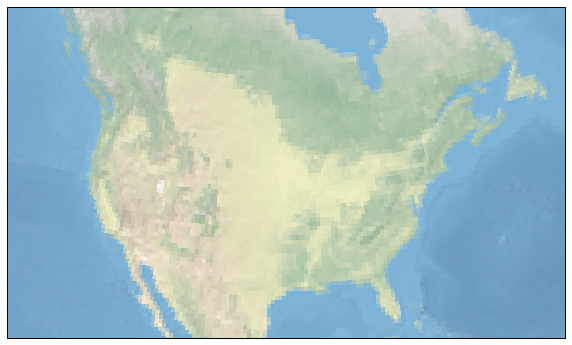### 2. Adding maps to CartoPy¶

CartoPy provides a couple helper methods for adding maps to the plot:

In :
fig = plt.figure(figsize=(10, 8))
ax = fig.add_subplot(1, 1, 1, projection=ccrs.LambertConformal())

ax.stock_img()

ax.set_extent([-130, -60, 20, 55])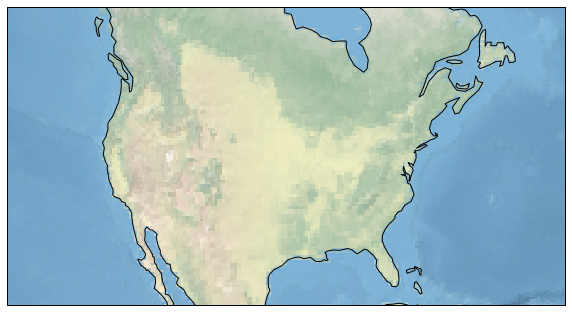Cartopy also has a lot of built-in support for a variety of map features:

In :
fig = plt.figure(figsize=(10, 8))
ax = fig.add_subplot(1, 1, 1, projection=ccrs.LambertConformal())

# Can also supply matplotlib kwargs

ax.set_extent([-130, -60, 20, 55])

/home/travis/miniconda/envs/unidata/lib/python3.7/site-packages/cartopy/io/__init__.py:260: DownloadWarning: Downloading: http://naciscdn.org/naturalearth/110m/physical/ne_110m_lakes.zip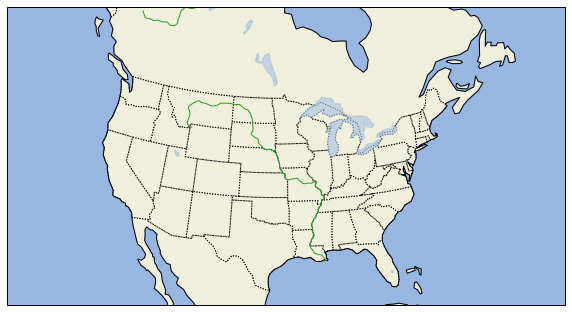The map features are available at several different scales depending on how large the area you are covering is. The scales can be accessed using the with_scale method. Natural Earth features are available at 110m, 50m and 10m.

In :
fig = plt.figure(figsize=(10, 8))
ax = fig.add_subplot(1, 1, 1, projection=ccrs.LambertConformal())

# Can also supply matplotlib kwargs

ax.set_extent([-130, -60, 20, 55])

/home/travis/miniconda/envs/unidata/lib/python3.7/site-packages/cartopy/io/__init__.py:260: DownloadWarning: Downloading: http://naciscdn.org/naturalearth/50m/physical/ne_50m_lakes.zip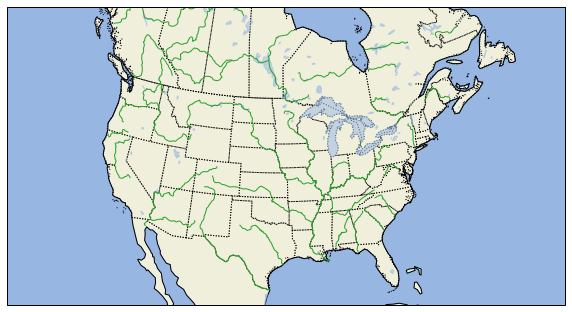You can also grab other features from the Natural Earth project: http://www.naturalearthdata.com/

### US Counties¶

MetPy has US Counties built in at the 20m, 5m, and 500k resolutions.

In :
from metpy.plots import USCOUNTIES

In :
proj = ccrs.LambertConformal(central_longitude=-85.0, central_latitude=45.0)

fig = plt.figure(figsize=(12, 9))
ax1 = fig.add_subplot(1, 3, 1, projection=proj)
ax2 = fig.add_subplot(1, 3, 2, projection=proj)
ax3 = fig.add_subplot(1, 3, 3, projection=proj)

for scale, axis in zip(['20m', '5m', '500k'], [ax1, ax2, ax3]):
axis.set_extent([270.25, 270.9, 38.15, 38.75], ccrs.Geodetic())

/home/travis/miniconda/envs/unidata/lib/python3.7/site-packages/pooch/core.py:379: UserWarning: Downloading data file 'us_counties_20m.dbf' from remote data store 'https://github.com/Unidata/MetPy/raw/v0.12.0/staticdata/us_counties_20m.dbf' to '/home/travis/.cache/metpy/v0.12.0'.
action_word[action], fname, self.get_url(fname), str(self.path)
action_word[action], fname, self.get_url(fname), str(self.path)
action_word[action], fname, self.get_url(fname), str(self.path)
action_word[action], fname, self.get_url(fname), str(self.path)
action_word[action], fname, self.get_url(fname), str(self.path)
action_word[action], fname, self.get_url(fname), str(self.path)
action_word[action], fname, self.get_url(fname), str(self.path)
action_word[action], fname, self.get_url(fname), str(self.path)
action_word[action], fname, self.get_url(fname), str(self.path)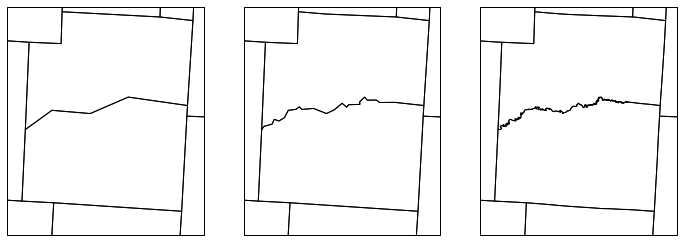### 3. Plotting Data¶

CartoPy supports all of the matplotlib plotting options you would expect on a map. It handles transforming your data between different coordinate systems transparently, provided you provide the correct information. (More on this later...). To start, let's put a marker at -105, 40:

In :
fig = plt.figure(figsize=(10, 8))
ax = fig.add_subplot(1, 1, 1, projection=ccrs.LambertConformal())

ax.plot(-105, 40, marker='o', color='tab:red')

ax.set_extent([-130, -60, 20, 55])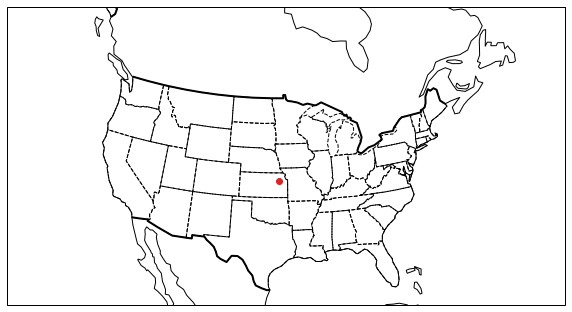So that did not succeed at putting a marker at -105 longitude, 40 latitude (Boulder, CO). Instead, what actually happened is that it put the marker at (-105, 40) in the map projection coordinate system; in this case that's a Lambert Conformal projection, and x,y are assumed in meters relative to the origin of that coordinate system. To get CartoPy to treat it as longitude/latitude, we need to tell it that's what we're doing. We do this through the use of the transform argument to all of the plotting functions.

In :
fig = plt.figure(figsize=(10, 8))
ax = fig.add_subplot(1, 1, 1, projection=ccrs.LambertConformal())

data_projection = ccrs.PlateCarree()
ax.plot(-105, 40, marker='o', color='tab:red', transform=data_projection)

ax.set_extent([-130, -60, 20, 55])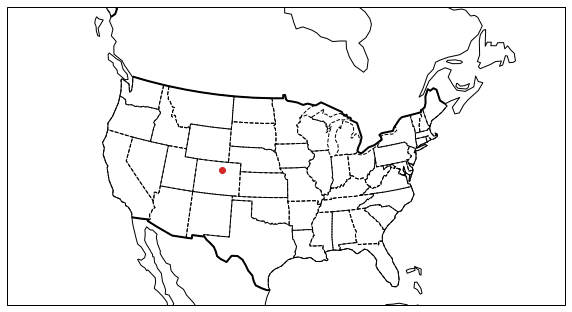This approach by CartoPy separates the data coordinate system from the coordinate system of the plot. It allows you to take data in any coordinate system (lon/lat, Lambert Conformal) and display it in any map you want. It also allows you to combine data from various coordinate systems seamlessly. This extends to all plot types, not just plot:

In :
# Create some synthetic gridded wind data
import numpy as np
from metpy.calc import wind_speed
from metpy.units import units

# Note that all of these winds have u = 0 -> south wind
v = (np.full((5, 5), 10, dtype=np.float64) + 10 * np.arange(5)) * units.knots
u = np.zeros_like(v) * units.knots
speed = wind_speed(u, v)

# Create arrays of longitude and latitude
x = np.linspace(-120, -60, 5)
y = np.linspace(30, 55, 5)

# Plot as normal
fig = plt.figure(figsize=(10, 8))
ax = fig.add_subplot(1, 1, 1, projection=ccrs.LambertConformal())

# Plot wind barbs--CartoPy handles reprojecting the vectors properly for the
# coordinate system
ax.barbs(x, y, u.m, v.m, transform=ccrs.PlateCarree(), color='tab:blue')

ax.set_extent([-130, -60, 20, 55])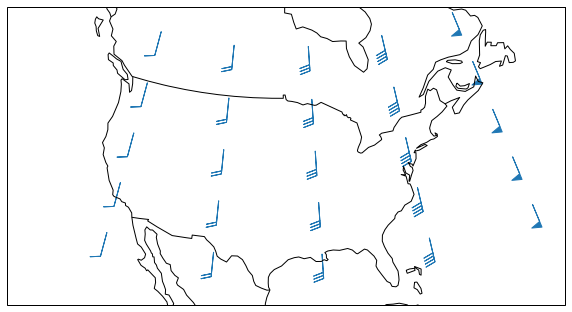EXERCISE:
• Create a map, on a Mercator Projection, which at least has coastlines and country and state borders. Bonus points for putting on colored land and oceans, or other map features.
• Plot our location correctly on the map.
• Set the bounds of the map to zoom in mostly over our state/region.
In :
# YOUR CODE GOES HERE

SOLUTION
In :
# %load solutions/map.py

# Cell content replaced by load magic replacement.
fig = plt.figure(figsize=(10, 8))
ax = fig.add_subplot(1, 1, 1, projection=ccrs.Mercator())